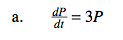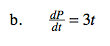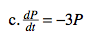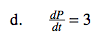### Home > CALC > Chapter 7 > Lesson 7.3.2 > Problem7-121

7-121.
1. Which of the following differential equations could model a population whose rate of change is proportional to the existing population? Explain your reasoning. Is it possible that there is more than one answer? Homework Help ✎

1.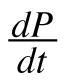= 3P

2.= 3t

3.= −3P

4.= 3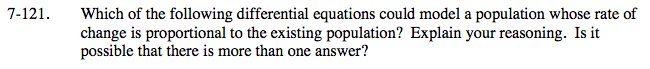A proportional situation follows the patten: y = kx.